# Trapezoidal Shape Volume (equal slopes at opposite sides) - Proof of Equations

In this trapezoidal volume calculations, the opposite sides have the same slopes. As the result, centers of bottom and top rectangles are at the same vertical line.

• Volume, V, in terms of D, W1, L1, W2, L2
• Volume, V, in terms of D, W1, L1, α , β
• Depth, D, in terms of V, W1, L1, α , β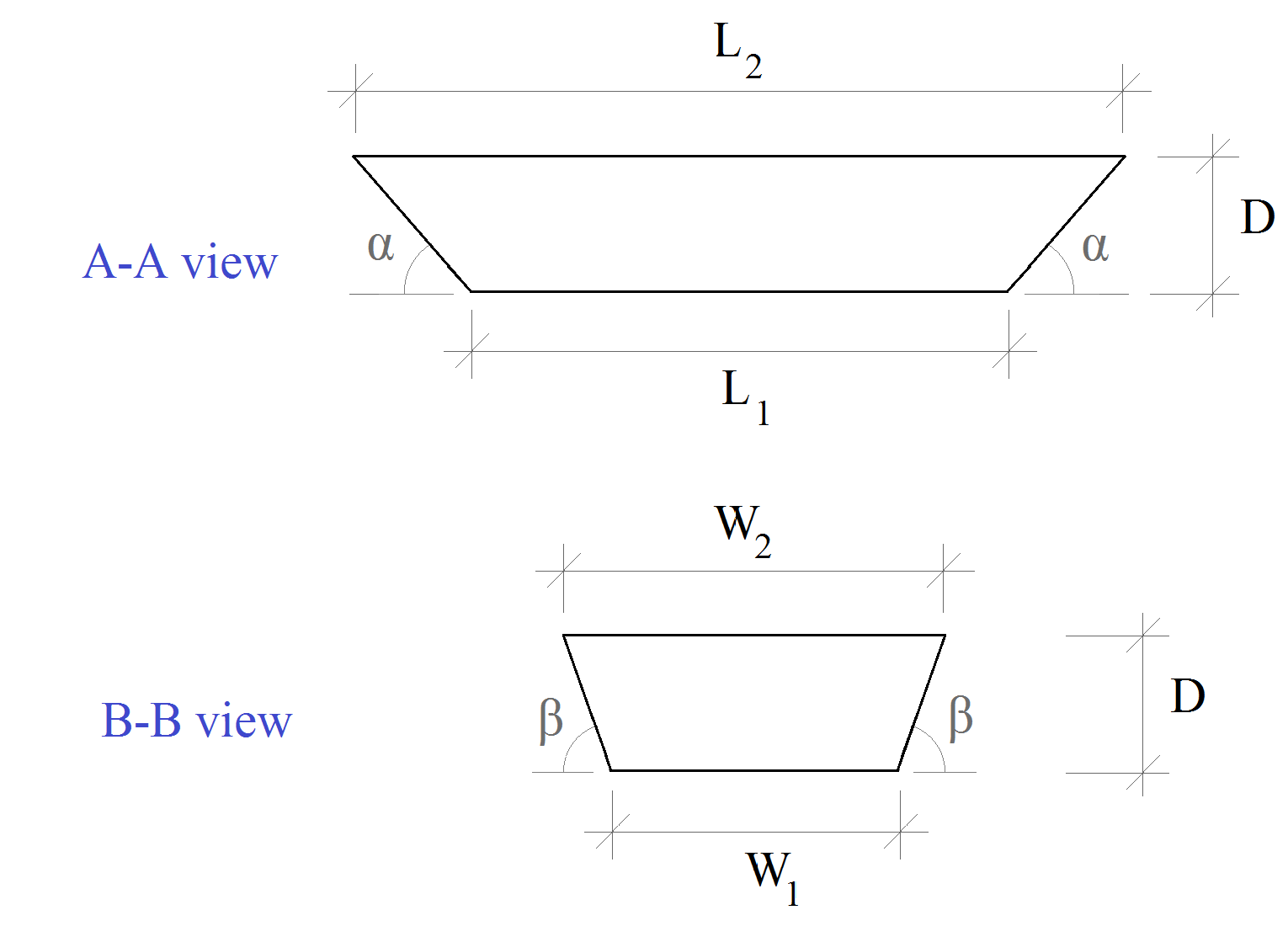L2 = L1
2D / tan α

W2 = W1
2D / tan β

In order to calculate the volume, the solid is divided into different sub-volumes:#### a) Center rectangular box, V1: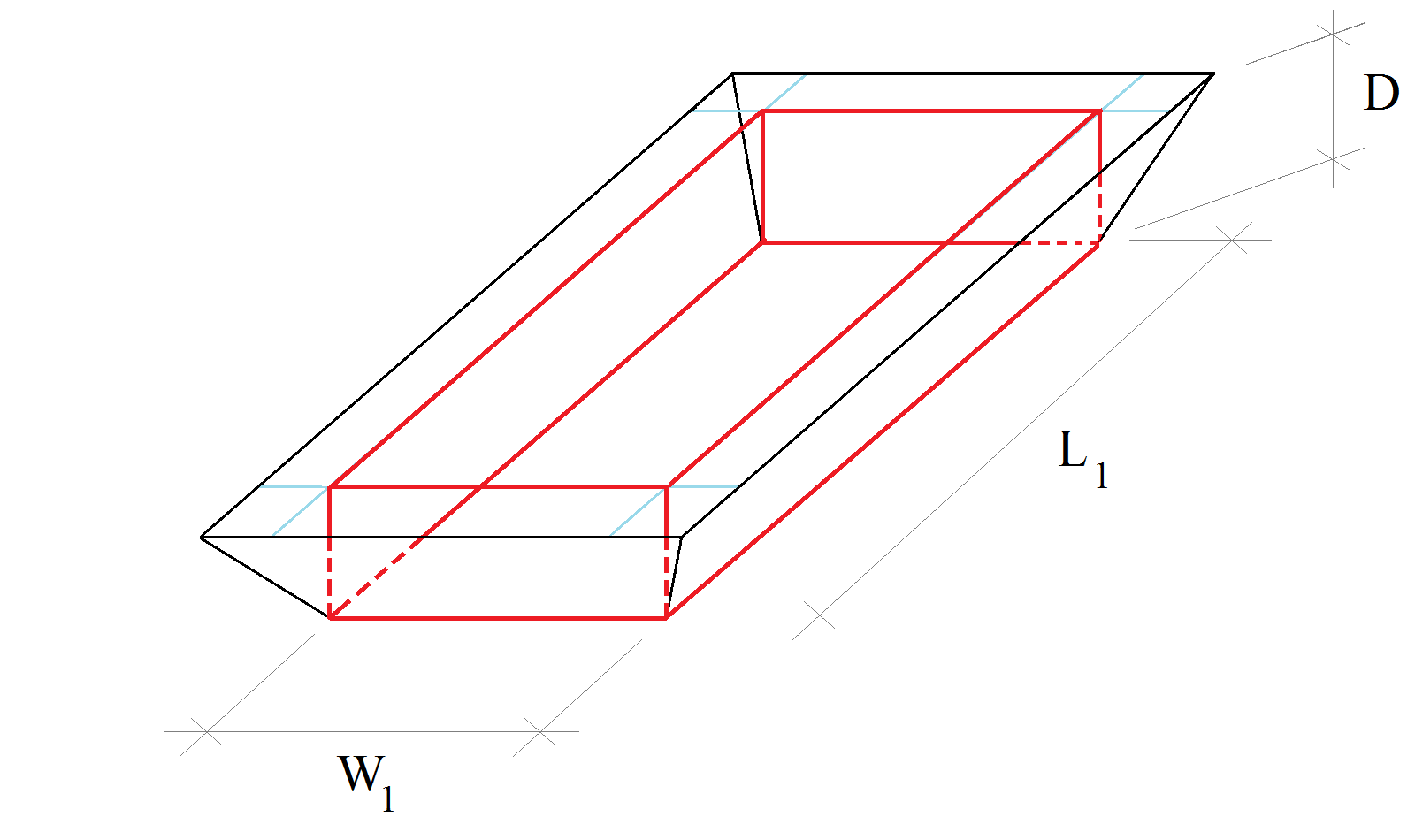V1 = W1 L1 D

#### b) Corner pyramids, V2: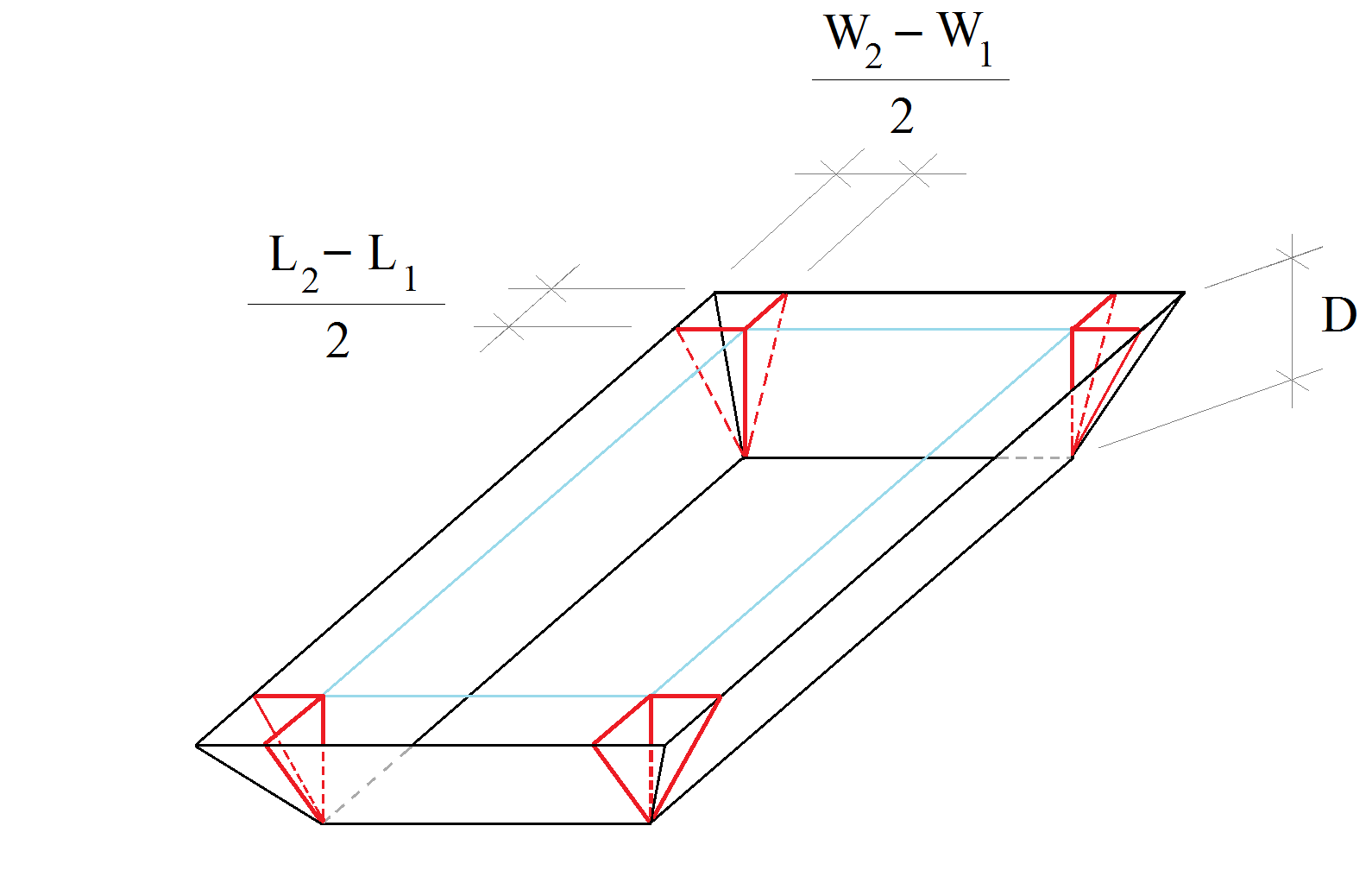V2 = 4 (corner pyramid volume) = 4 (
W2 – W1 / 2
) (
L2 – L1 / 2
) (
D / 3
)
V2 = (W2 – W1) (L2 – L1) (
D / 3
)

#### c) Side triangular prisms, V3: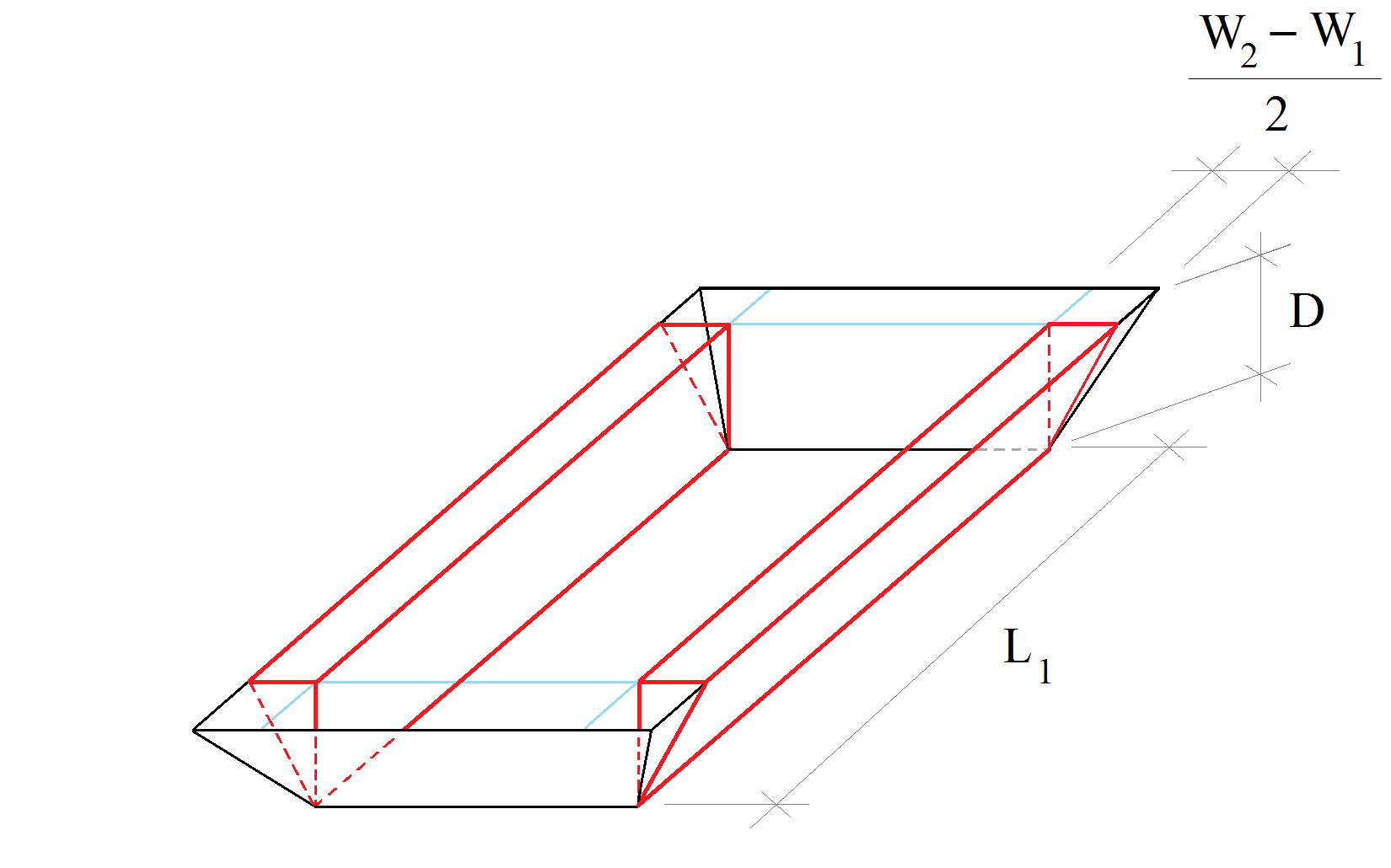V3 = 2 (side triangular prism volume) = 2 (
W2 – W1 / 2
) (
D / 2
) L1
V3 = (W2 – W1) (
D / 2
) L1

#### d) Side triangular prisms, V4: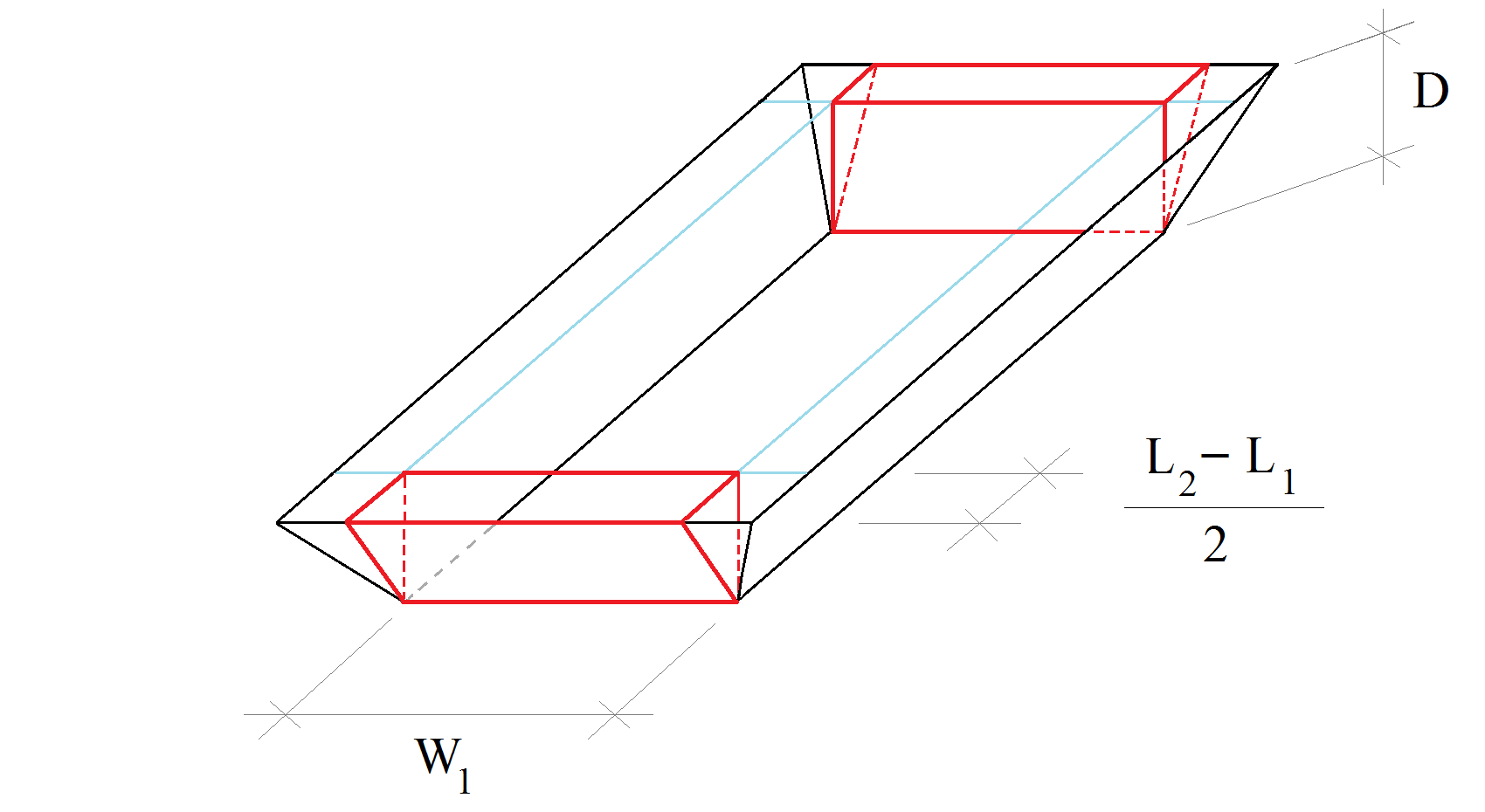V4 = 2 (side triangular prism volume) = 2 (
L2 – L1 / 2
) (
D / 2
) W1
V4 = (L2 – L1) (
D / 2
) W1

#### Trapezoidal volume, V, in terms of D, W1, L1, W2, L2:

V = V1 + V2 + V3 + V4
V = W1 L1 D + (W2 – W1) (L2 – L1) (
D / 3
) + (W2 – W1) (
D / 2
) L1 + (L2 – L1) (
D / 2
) W1
V = D [W1 L1 + (W2 – W1) (L2 – L1) (
1 / 3
) + (W2 – W1) (
1 / 2
) L1 + (L2 – L1) (
1 / 2
) W1 ]
V = D [W1 L1 + (
W2 L2  / 3
–
W2 L1  / 3
–
W1 L2  / 3
+
W1 L1  / 3
) + (
W2 L1  / 2
–
W1 L1  / 2
) + (
W1 L2  / 2
–
W1 L1  / 2
) ]
V = D [ W1 L1 (1 +
1 / 3
–
1 / 2
–
1 / 2
)  +
W2 L2  / 3
+ W2 L1 (
–1 / 3
+
1 / 2
)  + W1 L2 (
–1 / 3
+
1 / 2
) ]
V = D [
W1 L1  / 3
+
W2 L2  / 3
+
W2 L1  / 6
+
W1 L2  / 6
]
V =
D / 6
(2 W1 L1 + 2 W2 L2 + W2 L1 + W1 L2 )

#### Trapezoidal volume, V, in terms of D, W1, L1, α , β :

V =
D / 6
(2 W1 L1 + 2 W2 L2 + W2 L1 + W1 L2 )
Substitute W2, L2 with:      W2 = W1
2D / tan β
,   L2 = L1
2D / tan α

V =
D / 6
[2 W1 L1 + 2 (W1
2D / tan β
) (L1
2D / tan α
) + (W1
2D / tan β
) L1 + W1 (L1
2D / tan α
) ]
V =
D / 6
[2 W1 L1 + 2 (W1 L1
2W1 / tan α
D +
2L1 / tan β
D +
4 / (tan α)(tan β)
D2 ) + W1 L1  +
2L1 / tan β
D + W1 L1  +
2W1 / tan α
D ]
V =
D / 6
(2 W1 L1 + 2 W1 L1
4W1 / tan α
D +
4L1 / tan β
D +
8 / (tan α)(tan β)
D2 + W1 L1  +
2L1 / tan β
D + W1 L1  +
2W1 / tan α
D )
V =
D / 6
[6 W1 L1 + (
6W1 / tan α
+
6L1 / tan β
) D +
8 / (tan α)(tan β)
D2 ]
V = W1 L1 D + (
W1 / tan α
+
L1 / tan β
) D2
4 / 3 (tan α)(tan β)
D3

#### Depth, D, in terms of V, W1, L1, α , β :

V = W1 L1 D + (
W1 / tan α
+
L1 / tan β
) D2
4 / 3 (tan α)(tan β)
D3

Writing this equation in cubic polynomial form:
4 / 3 (tan α)(tan β)
D3  + (
W1 / tan α
+
L1 / tan β
) D2 + W1 L1 D – V = 0

x = D
a =
4 / 3 (tan α)(tan β)

b =
W1 / tan α
+
L1 / tan β

c = W1 L1
d = – V

a x3 + b x2 + c x + d = 0

This equation has at least one solution amongst real numbers, and it can be found using the following equations: (Wikipedia Cubic Function)
Δ0 = b2 – 3ac
Δ1 = 2b3 – 9abc + 27a2d
C = (
Δ1 + (Δ12 – 4Δ03)½ / 2
)
x =
–1 / 3a
(b + C +
Δ0 / C
)

Disclaimer:
I do not claim in any way that these calculators are free of errors - I develop them as a hobby - so use them at your own discretion. If you encounter an error, please let me know here.